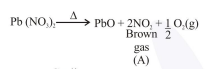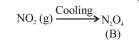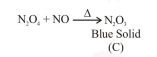# On heating, lead(II) nitrate gives a brown gas (A).

Question:

On heating, lead(II) nitrate gives a brown gas (A). The gas (A) on cooling changes to a colourless solid/liquid (B). (B) on heating with $\mathrm{NO}$

changes to a blue solid $(\mathrm{C})$. The oxidation number of nitrogen in solid (C) is :

1. $+5$

2. $+2$

3. $+4$

4. +3

Correct Option: , 4

Solution:O.S. of nitrogen in $\mathrm{N}_{2} \mathrm{O}_{3}$ is $+3$

$\mathrm{N}_{2} \mathrm{O}_{3} 2 \mathrm{x}+3(-2)=0$

$x=+3$